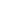Flat 50% off

Ends in

## In the Young's double slit experiment, the intensities at two points $P_{1}$ and $P_{2}$ on the screen are respectively $I_{1}$ and $I_{2}$. If $P_{1}$ is located at the centre of a bright fringe and $P_{2}$ is located at a distance euqal to a quarter of fringe width from $P_{1}$, then $\frac{I_{1}}{I_{2}}$ is(a) 2(b) $\frac{1}{2}$(c) 4(d) 16

### Asked By kona cheran kumar

Updated Mon, 27 May 2019 05:16 pm